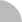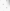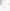# 臺灣博碩士論文加值系統

(44.192.22.242) 您好！臺灣時間：2021/07/31 09:36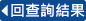:::

### 詳目顯示

: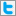Twitter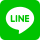•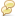被引用:0
•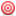點閱:107
•評分: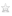•下載:7
•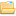書目收藏:0
 在本篇論文中，我們主要研究二維穩態不可壓縮那維爾-史托克問題在給定速度邊界值情況下的最小平方有限元素法之片狀線性數值解。首先引入兩個新未知函數〝旋度和總壓力〞後，將那維爾-史托克問題分別改寫成〝速度-旋度-壓力〞和〝速度-旋度-總壓力〞兩個一階偏微分方程組。接著我們引用L2最小平方與網格依賴加權最小平方有限元素法求解源自於皮卡型迭代法所產生的系列奧辛問題。其中相對應的最小平方能量泛函定義在一個適當乘積函數空間下，分別取每項偏微分方程式殘餘的L2模未加權或加權之平方和。數值實驗證明，在雷諾數小的時候，L2最小平方法比網格依賴加權最小平方法更準確；然而對大雷諾數的問題，網格依賴加權最小平方法比L2最小平方法明顯地好很多。最後列出在不同雷諾數下凹槽驅動流場的數值結果。
 In this thesis, we study the piecewise bilinear finite element approximations to the two-dimensional stationary incompressible Navier-Stokes equations with the velocity boundary condition by using the least-squares principles.The Navier-Stokes problem is first recast into the velocity-vorticity-pressure and velocity-vorticity-total pressure first-order systems by introducing the vorticity variable and, in addition, total pressure variable. We then apply both the L2 least-squares and mesh-dependent weighted least-squares finite element schemesto approximate the solutions of the sequence of Oseen problems arising from a Picard-type iteration associated with these first-order systems. The corresponding least-squares energy functionals are defined in terms of the sum of the squared L2 norms without or with mesh-dependent weights of the residual equations over a product function space. Numerical evidences show that, for low Reynolds number flows, the L2 least-squares method is more accurate than the mesh-dependent weighted least-squares method.For flows with large Reynolds numbers, the mesh-dependent weighted least-squares method is apparently better than the L2 least-squares method. Some numerical results for driven cavity flows with various Reynolds numbers are also given.
 Abstract 11. Problem formulation 22. Least-squares finite element methods 53. Some theoretical results for the Oseen problem 94. Numerical results for smooth exact solutions 105. Numerical results for driven cavity flows 186. Conclusions 44References 45
  P. B. Bochev, Analysis of least-squares finite element methods for theNavier-Stokes equations, SIAM, J. Numer. Anal., 34 (1997), pp. 1817-1844. P. B. Bochev and M. D. Gunzburger, Analysis of least-squares finiteelement methods for the Stokes equations, Math. Comp., 63 (1994),pp 479-506. P. B. Bochev and M. D. Gunzburger, Finite element methods of least-squarestype, SIAM Rev., 40 (1998), pp 789-837. P. B. Bochev, Z. Cai, T. A. Manteuffel and S. F. McCormick, Analysis ofvelocity-flux first-order system least-squares principles for the Navier-Stokes equations: Part I, SIAM J. Numer. Anal., 35 (1998), pp. 990-1009. S. C. Brenner and L. R. Scott, The Mathematical Theory of Finite ElementMethods, Springer-Verlag, New York, 1994. F. Brezzi and M. Fortin, Mixed and Hybrid Finite Element Methods,Springer-Verlag, New York, 1991. Z. Cai, T. Manteuffel, and S. McCormick, First-order system least squaresfor velocity-vorticity-pressure form of the Stokes equations, withapplication to linear elasticity, ETNA, 3 (1995), pp. 150-159. Z. Cai, T. A. Manteuffel, and S. F. McCormick, First-order system leastsquares for the Stokes equations, with application to linear elasticity,SIAM J. Numer. Anal., 34 (1997), pp. 1727-1741. C. L. Chang, An error estimate of the least squares finite elementmethod for the Stokes problem in three dimensions, Math. Comp., 63 (1994),pp. 41-50. C. L. Chang and B.-N. Jiang, An error analysis of least-squares finiteelement method of velocity-pressure-vorticity formulation for Stokesproblem, Comput. Methods Appl. Mech. Engrg., 84 (1990), pp. 247-255. C. L. Chang and J. J. Nelson, Least-squares finite element method for theStokes problem with zero residual of mass conservation, SIAM J. Numer.Anal., 34 (1997), pp. 480-489. C. L. Chang, S.-Y. Yang, and C.-H. Hsu, A least-squares finite elementmethod for incompressible flow in stress-velocity-pressure version,Comput. Methods Appl. Mech. Engrg., 128 (1995), pp. 1-9. C. L. Chang and S.-Y. Yang, Analysis of the L2 least-squares finiteelement method for the velocity-vorticity-pressure Stokes equationswith velocity boundary conditions, Appl. Math. Comput., 130 (2002),pp. 121-144. J. M. Deang and M. D. Gunzburger, Issues related to least-squares finiteelement methods for the Stokes equations, 20 (1998), pp. 878-906. H.-Y. Duan and G.-P. Liang, On the velocity-pressure-vorticity least-squares mixed finite element method for the 3D Stokes equations,SIAM J. Numer. Anal., 41 (2003), pp. 2114-2130. M. Feistauer, Mathematical Methods in Fluid Dynamics, Longman Group UKLimited, 1993. V. Girault and P. A. Raviart, Finite Element Methods for Navier-StokesEquations: Theory and Algorithms, Springer-Verlag, New York, 1986. B.-N. Jiang, The Least-Squares Finite Element Method, Springer-Verlag,Berlin, 1998. S. D. Kim, Y. H. Lee, and S.-Y. Yang, Analysis of [H(-1), L2, L2]first-order system least squares for the incompressible Oseen typeequations, to appear in Appl. Numer. Math. C.-C. Tsai and S.-Y. Yang, On the velocity-vorticity-pressure least-squares finite element method for the stationary incompressible Oseenproblem, submitted for publication, 2004. S.-Y. Yang, Error analysis of a weighted least-squares finiteelement method for 2-D incompressible flows in velocity-stress-pressureformulation, Math. Meth. Appl. Sci., 21 (1998), pp. 1637-1654.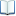電子全文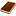國圖紙本論文推文當script無法執行時可按︰推文 網路書籤當script無法執行時可按︰網路書籤 推薦當script無法執行時可按︰推薦 評分當script無法執行時可按︰評分 引用網址當script無法執行時可按︰引用網址 轉寄當script無法執行時可按︰轉寄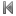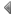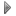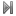top
 1 兩種迭代最小平方有限元素法求解不可壓縮那維爾-史托克方程組之研究

 1 郭崑謨、闕河士(1990)，顧客抱怨行為及其影響因素之研究，管理評論，7月，頁155-173。

 1 兩種迭代最小平方有限元素法求解不可壓縮那維爾-史托克方程組之研究 2 二維穩態不可壓縮磁流體問題的迭代最小平方有限元素法之數值計算 3 以奈維爾-史托克方程式與等位函數法求解自由液面流場之研究 4 高階橢圓偏微分方程解的存在性及其行為之研究 5 含圓形邊界史托克斯流與板問題之半解析法 6 Global Exponential Stability of Modified RTD-based Two-Neuron Networks with Discrete Time Delays 7 在N維實數域之雙調和微分方程 8 以速度-渦度與有限元素法解析三維奈維爾-史托克斯方程式 9 壓電式振動陀螺儀之三維有限元素分析 10 新金融秩序下金融控股公司監理法制之再建構 11 不可壓縮Navier-Stokes方程式的緊密且精準的差分表示法之數值測試：唇拉方穴流 12 美國聯邦民事訴訟程序法有關證據開示程序之研究—以電子化儲存資訊為中心 13 專門職業及技術人員考試委託民間辦理法制面之研究 14 基於ABAQUS之功能梯度材料旋轉圓盤有限元素分析 15 三相感應機於史坦梅茲接法下之平衡操作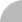簡易查詢 | 進階查詢 | 熱門排行 | 我的研究室